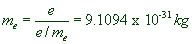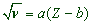# SUB ATOMIC PARTICLES

* Protons, neutrons and electrons are known as sub-atomic particles.

* Protons and neutrons are are found at the tiny central part of the atom - called nucleus and hence are collectively called as nucleons. The nucleus contributes most of the mass to atom. It is positively charged due to presence of protons.

* Whereas, negatively charged electrons are found revolving around the nucleus.## ELECTRON

Electron: The negatively charged fundamental particle present in an atom with negligible mass is called electron.

The mass of an electron is 1/1836 of mass of proton or hydrogen atom. It is equal to 0.000548 amu or 9.1095X10-31 kg. Its charge is 1.6022X10-19 coulomb.

Discovery of Electron: Sir William Crookes designed a cathode ray discharge tube. This is made up of a sealed glass tube filled with a specific gas and consists of two metallic electrodes i.e. a cathode and an anode at the opposite ends, which are connected a high voltage source of electricity. The discharge tube is connected to a vacuum pump, which is used to reduce the pressure inside by evacuating the gas.

It was observed that a stream of particle rays are produced from cathode upon applying high voltage at reduced pressures. These are known as cathode rays. The particles of the rays are negatively charged and are now known as electrons.Characteristics of cathode rays :

1) Cathode rays are not visible but their behavior can be observed with the help of a fluorescent or a phosphorescent.

2) These rays travel from cathode to anode.

3) These rays travel in straight lines in the absence of electric and magnetic fields.

4) However these rays are deflected towards the positive end in electric field. Hence it was concluded that the rays constitute negatively charged particles and are known as electrons.

These rays are also deflected in magnetic field.

5) The behavior and the properties of rays are independent of the nature of the cathode material and nature of the gas present in the cathode ray tube

These facts conclude that the electrons are the negatively charged fundamental particles present in all the substances.

Charge to mass ratio of Electron (e/me): The charge to mass ratio of electron was calculated by J. J. Thomson. Its value is equal to 1.75882x1011C.kg-1.

Charge on the Electron (e) : The charge on the electron was calculated by Millikan in oil drop experiment as 1.60 x 10-19 coulombs.

Now the mass of an electron can be derived as follows:## PROTON

Proton: The positively charged fundamental particle present in the atom is called proton.

* The mass of a proton is 1.007277 amu or 1.67252 X 10-27 kg.

* Its charge is same as that of electron i.e., 1.60 x 10-19 coulombs.

Discovery of proton : Protons are discovered in canal ray experiment. These rays are produced in modified cathode ray tube.

Characteristics of canal rays:

1) The Canal rays are produced from the anode and travel towards the cathode. They constitute positively charged particles.

2) The characteristics of these rays depend on the nature of gas present in the cathode ray tube

E.g. The e/m ratio of the particles depend on the nature of gas taken.

3) Some of the positively charged particles carry a multiple of the fundamental unit of electrical charge.

4) The behavior of these particles in the magnetic or electric field is opposite to that observed for electron. They are deflected towards the negative end of the electric field.

6) When hydrogen gas is used in the discharge tube, the positively charged particles emitted are found to possess mass of 1 amu and these are called protons.

## NEUTRON

Neutron: The neutral fundamental particle present in the atom is called neutron.

* The mass of a neutron is 1.00898 amu or 1.67495 X 10-27kg.

* It has no charge.

Discovery of neutrons: Chadwick discovered neutrons during the bombardment of a thin layer of 4Be9 with α-particles.

 Particle Charge Relative charge Mass in amu Mass in kg Electron -1.602 x 10-19 -1 0.000542 amu 9.1 x 10-31 kg Proton +1.602 x 10-19 +1 1.00727 amu 1.672 x 10-27 kg Neutron 0 0 1.00867 amu 1.674 x 10-27 kg

## ATOMIC NUMBER, MASS NUMBER, ISOTOPES, ISOBARS, ATOMIC WEIGHT

Atomic number (Z):The number of protons or the number of electrons in an atom is called atomic number. It is represented by 'Z'.

Moseley discovered a simple relation between the frequencies of the characteristic X-rays of an element and its atomic number.ν = frequency of X-rays

Z = atomic number

a & b are constants which are characteristic of elements

Mass number(A): The total number of protons and neutrons in an atom is called mass number. It is denoted by 'A'.

A = no. of protons + no. of neutrons

A = Z + no. of neutrons no. of neutrons = A - Z

Isotope: Isotopes are the atoms of an element with same atomic number but differ in their mass numbers i.e., the isotopes of an element have same number of protons but differ in the number of neutrons.

E.g. Hydrogen has three isotopes :

Hydrogen(1H1),

Deuterium (1H2) and

Tritium (1H3).

They have same number of protons (= 1) but the numbers of neutrons are 1, 2 and 3 respectively.

Isobars : The atoms of different elements with same mass number but different atomic numbers are called “Isobars”.

E.g. 6C14, 7N14

Atomic weight: The atomic weight of an element is the average weight of all the isotopes of that element. It is usually expressed as gram atomic weight.

Note: The atomic number is a whole number whereas the atomic weight may be a fractional number.

 Atomic structure: TOC Early Atomic models >